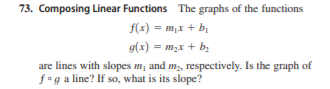# 73. Composing Linear Functions The graphs of the functions f(x) = m,x + bị g(x) = m,x + b. %3D are lines with slopes m, and m,, respectively. Is the graph of f°g a line? If so, what is its slope?

Question

Composing Linear Functions The graphs of the functions
f1x2  m1x  b1
g1x2  m2 x  b2
are lines with slopes m1 and m2, respectively. Is the graph of
f  g a line? If so, what is its slope?help_outlineImage Transcriptionclose73. Composing Linear Functions The graphs of the functions f(x) = m,x + bị g(x) = m,x + b. %3D are lines with slopes m, and m,, respectively. Is the graph of f°g a line? If so, what is its slope? fullscreen

### Want to see this answer and more?

Experts are waiting 24/7 to provide step-by-step solutions in as fast as 30 minutes!*

*Response times may vary by subject and question complexity. Median response time is 34 minutes for paid subscribers and may be longer for promotional offers.
Tagged in
Math
Algebra

### Other